# 2 data structure in R

3 de Oct de 2016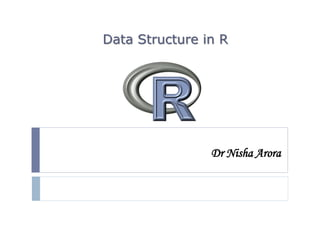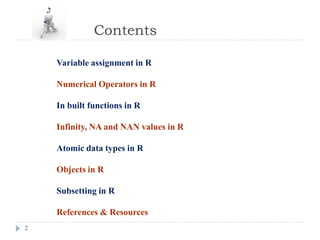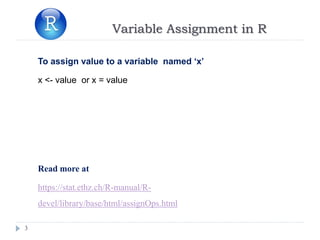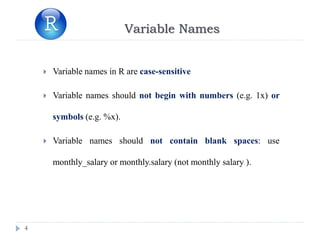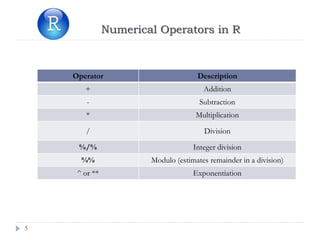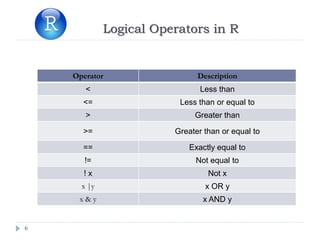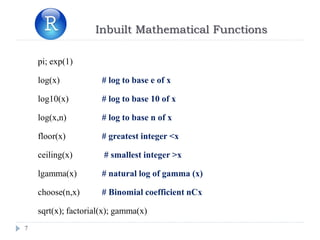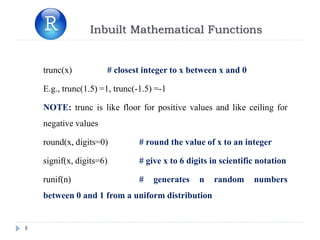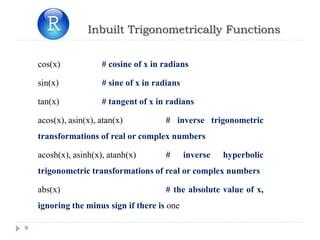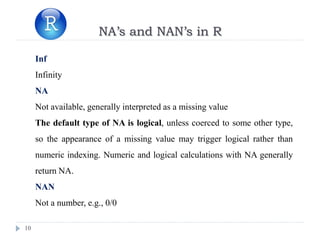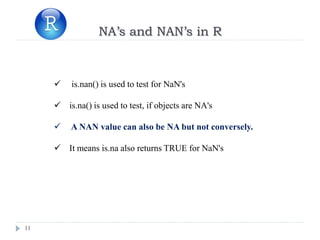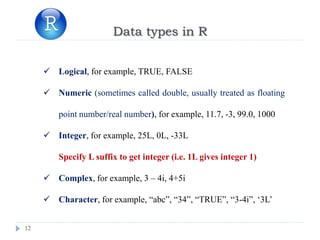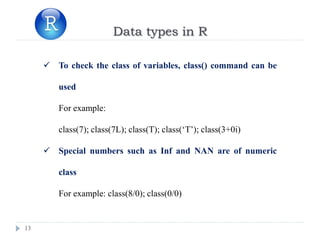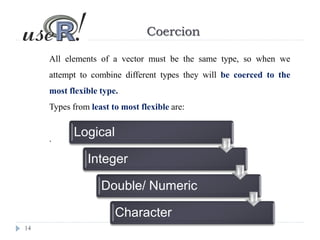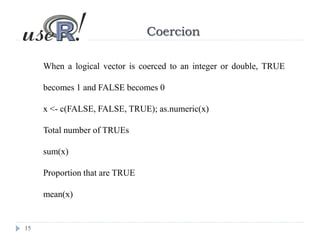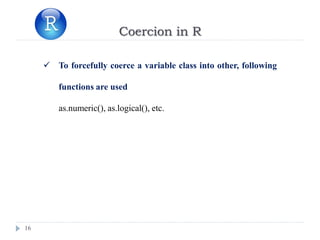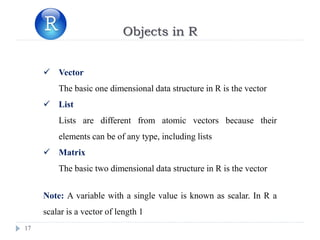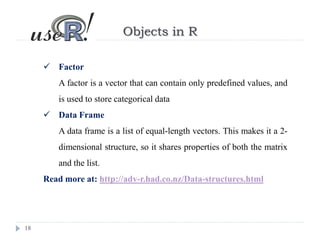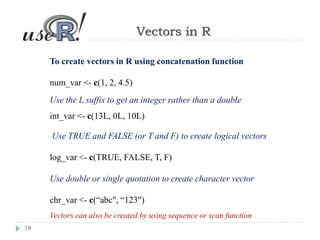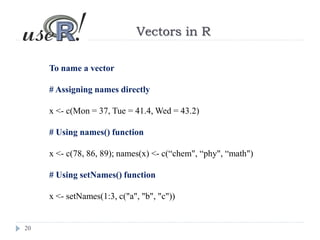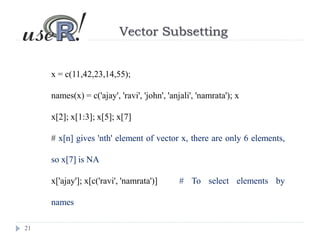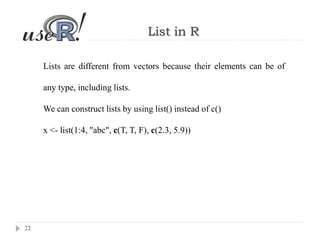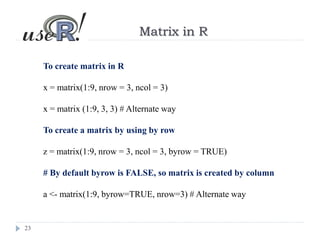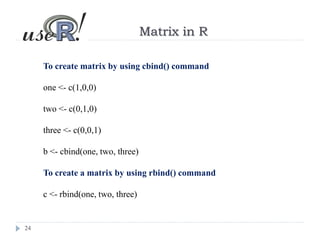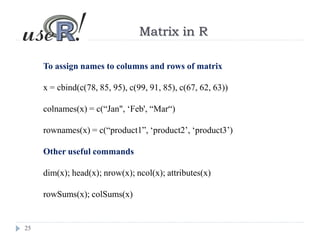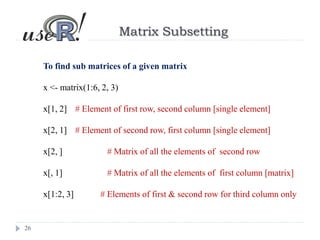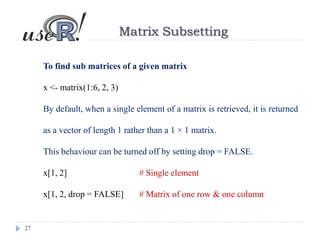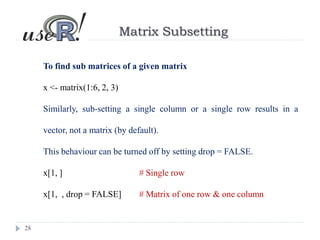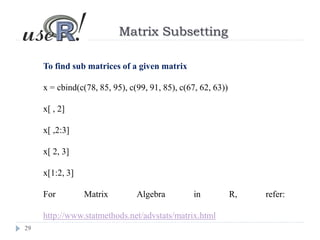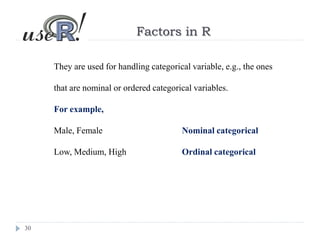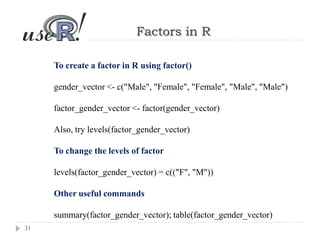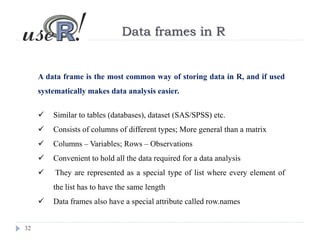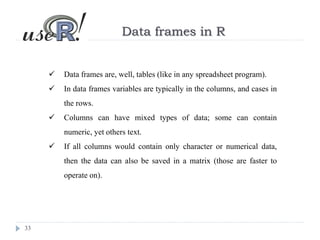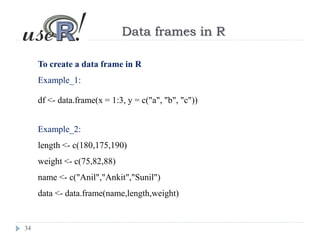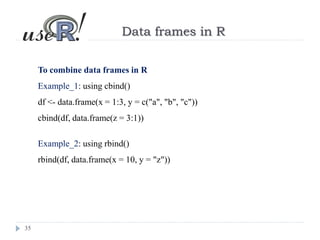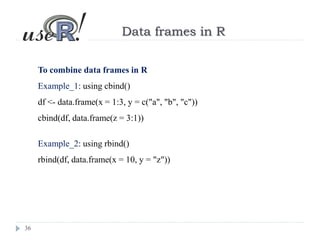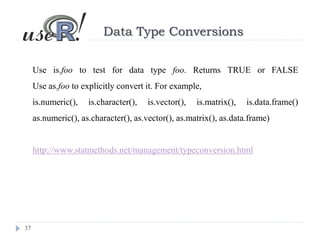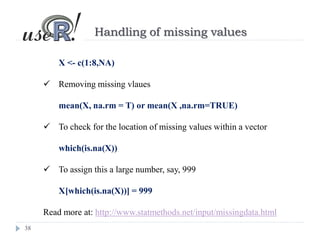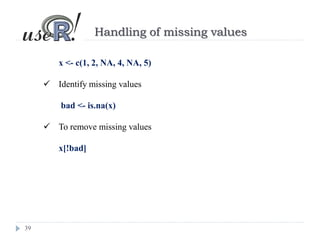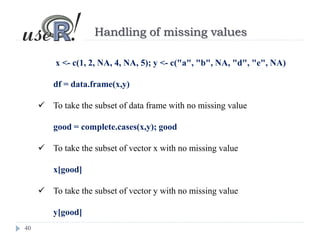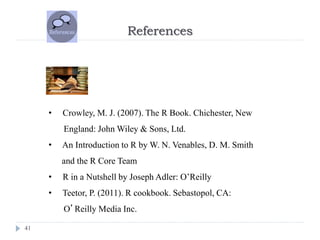1 de 45

### 2 data structure in R

1. Dr Nisha Arora Data Structure in R
2. Contents 2 Variable assignment in R Numerical Operators in R In built functions in R Infinity, NA and NAN values in R Atomic data types in R Objects in R Subsetting in R References & Resources
3. Variable Assignment in R 3 To assign value to a variable named ‘x’ x <- value or x = value x <<- value value -> x value ->> x Read more at https://stat.ethz.ch/R-manual/R- devel/library/base/html/assignOps.html
4. Variable Names 4  Variable names in R are case-sensitive  Variable names should not begin with numbers (e.g. 1x) or symbols (e.g. %x).  Variable names should not contain blank spaces: use monthly_salary or monthly.salary (not monthly salary ).
5. Numerical Operators in R 5 Operator Description + Addition - Subtraction * Multiplication / Division %/% Integer division %% Modulo (estimates remainder in a division) ^ or ** Exponentiation
6. Logical Operators in R 6 Operator Description < Less than <= Less than or equal to > Greater than >= Greater than or equal to == Exactly equal to != Not equal to ! x Not x x |y x OR y x & y x AND y
7. Inbuilt Mathematical Functions 7 pi; exp(1) log(x) # log to base e of x log10(x) # log to base 10 of x log(x,n) # log to base n of x floor(x) # greatest integer <x ceiling(x) # smallest integer >x lgamma(x) # natural log of gamma (x) choose(n,x) # Binomial coefficient nCx sqrt(x); factorial(x); gamma(x)
8. Inbuilt Mathematical Functions 8 trunc(x) # closest integer to x between x and 0 E.g., trunc(1.5) =1, trunc(-1.5) =-1 NOTE: trunc is like floor for positive values and like ceiling for negative values round(x, digits=0) # round the value of x to an integer signif(x, digits=6) # give x to 6 digits in scientific notation runif(n) # generates n random numbers between 0 and 1 from a uniform distribution
9. Inbuilt Trigonometrically Functions 9 cos(x) # cosine of x in radians sin(x) # sine of x in radians tan(x) # tangent of x in radians acos(x), asin(x), atan(x) # inverse trigonometric transformations of real or complex numbers acosh(x), asinh(x), atanh(x) # inverse hyperbolic trigonometric transformations of real or complex numbers abs(x) # the absolute value of x, ignoring the minus sign if there is one
10. 10 NA’s and NAN’s in R Inf Infinity NA Not available, generally interpreted as a missing value The default type of NA is logical, unless coerced to some other type, so the appearance of a missing value may trigger logical rather than numeric indexing. Numeric and logical calculations with NA generally return NA. NAN Not a number, e.g., 0/0
11. 11 NA’s and NAN’s in R  is.nan() is used to test for NaN's  is.na() is used to test, if objects are NA's  A NAN value can also be NA but not conversely.  It means is.na also returns TRUE for NaN's
12. 12 Data types in R  Logical, for example, TRUE, FALSE  Numeric (sometimes called double, usually treated as floating point number/real number), for example, 11.7, -3, 99.0, 1000  Integer, for example, 25L, 0L, -33L Specify L suffix to get integer (i.e. 1L gives integer 1)  Complex, for example, 3 – 4i, 4+5i  Character, for example, “abc”, “34”, “TRUE”, “3-4i”, ‘3L’
13. 13 Data types in R  To check the class of variables, class() command can be used For example: class(7); class(7L); class(T); class(‘T’); class(3+0i)  Special numbers such as Inf and NAN are of numeric class For example: class(8/0); class(0/0)
14. 14 Coercion All elements of a vector must be the same type, so when we attempt to combine different types they will be coerced to the most flexible type. Types from least to most flexible are: . Logical Integer Double/ Numeric Character
15. 15 Coercion When a logical vector is coerced to an integer or double, TRUE becomes 1 and FALSE becomes 0 x <- c(FALSE, FALSE, TRUE); as.numeric(x) Total number of TRUEs sum(x) Proportion that are TRUE mean(x)
16. 16 Coercion in R  To forcefully coerce a variable class into other, following functions are used as.numeric(), as.logical(), etc.
17. 17 Objects in R  Vector The basic one dimensional data structure in R is the vector  List Lists are different from atomic vectors because their elements can be of any type, including lists  Matrix The basic two dimensional data structure in R is the vector Note: A variable with a single value is known as scalar. In R a scalar is a vector of length 1
18. 18 Objects in R  Factor A factor is a vector that can contain only predefined values, and is used to store categorical data  Data Frame A data frame is a list of equal-length vectors. This makes it a 2- dimensional structure, so it shares properties of both the matrix and the list. Read more at: http://adv-r.had.co.nz/Data-structures.html
19. 19 Vectors in R To create vectors in R using concatenation function num_var <- c(1, 2, 4.5) Use the L suffix to get an integer rather than a double int_var <- c(13L, 0L, 10L) Use TRUE and FALSE (or T and F) to create logical vectors log_var <- c(TRUE, FALSE, T, F) Use double or single quotation to create character vector chr_var <- c(“abc", “123") Vectors can also be created by using sequence or scan function
20. 20 Vectors in R To name a vector # Assigning names directly x <- c(Mon = 37, Tue = 41.4, Wed = 43.2) # Using names() function x <- c(78, 86, 89); names(x) <- c(“chem", “phy", “math") # Using setNames() function x <- setNames(1:3, c("a", "b", "c"))
21. 21 Vector Subsetting x = c(11,42,23,14,55); names(x) = c('ajay', 'ravi', 'john', 'anjali', 'namrata'); x x; x[1:3]; x; x # x[n] gives 'nth' element of vector x, there are only 6 elements, so x is NA x['ajay']; x[c('ravi', 'namrata')] # To select elements by names
22. 22 List in R Lists are different from vectors because their elements can be of any type, including lists. We can construct lists by using list() instead of c() x <- list(1:4, "abc", c(T, T, F), c(2.3, 5.9))
23. 23 Matrix in R To create matrix in R x = matrix(1:9, nrow = 3, ncol = 3) x = matrix (1:9, 3, 3) # Alternate way To create a matrix by using by row z = matrix(1:9, nrow = 3, ncol = 3, byrow = TRUE) # By default byrow is FALSE, so matrix is created by column a <- matrix(1:9, byrow=TRUE, nrow=3) # Alternate way
24. 24 Matrix in R To create matrix by using cbind() command one <- c(1,0,0) two <- c(0,1,0) three <- c(0,0,1) b <- cbind(one, two, three) To create a matrix by using rbind() command c <- rbind(one, two, three)
25. 25 Matrix in R To assign names to columns and rows of matrix x = cbind(c(78, 85, 95), c(99, 91, 85), c(67, 62, 63)) colnames(x) = c(“Jan", ‘Feb', “Mar“) rownames(x) = c(“product1”, ‘product2’, ‘product3’) Other useful commands dim(x); head(x); nrow(x); ncol(x); attributes(x) rowSums(x); colSums(x)
26. 26 Matrix Subsetting To find sub matrices of a given matrix x <- matrix(1:6, 2, 3) x[1, 2] # Element of first row, second column [single element] x[2, 1] # Element of second row, first column [single element] x[2, ] # Matrix of all the elements of second row x[, 1] # Matrix of all the elements of first column [matrix] x[1:2, 3] # Elements of first & second row for third column only
27. 27 Matrix Subsetting To find sub matrices of a given matrix x <- matrix(1:6, 2, 3) By default, when a single element of a matrix is retrieved, it is returned as a vector of length 1 rather than a 1 × 1 matrix. This behaviour can be turned off by setting drop = FALSE. x[1, 2] # Single element x[1, 2, drop = FALSE] # Matrix of one row & one column
28. 28 Matrix Subsetting To find sub matrices of a given matrix x <- matrix(1:6, 2, 3) Similarly, sub-setting a single column or a single row results in a vector, not a matrix (by default). This behaviour can be turned off by setting drop = FALSE. x[1, ] # Single row x[1, , drop = FALSE] # Matrix of one row & one column
29. 29 Matrix Subsetting To find sub matrices of a given matrix x = cbind(c(78, 85, 95), c(99, 91, 85), c(67, 62, 63)) x[ , 2] x[ ,2:3] x[ 2, 3] x[1:2, 3] For Matrix Algebra in R, refer: http://www.statmethods.net/advstats/matrix.html
30. 30 Factors in R They are used for handling categorical variable, e.g., the ones that are nominal or ordered categorical variables. For example, Male, Female Nominal categorical Low, Medium, High Ordinal categorical
31. 31 Factors in R To create a factor in R using factor() gender_vector <- c("Male", "Female", "Female", "Male", "Male") factor_gender_vector <- factor(gender_vector) Also, try levels(factor_gender_vector) To change the levels of factor levels(factor_gender_vector) = c(("F", "M")) Other useful commands summary(factor_gender_vector); table(factor_gender_vector)
32. 32 Data frames in R A data frame is the most common way of storing data in R, and if used systematically makes data analysis easier.  Similar to tables (databases), dataset (SAS/SPSS) etc.  Consists of columns of different types; More general than a matrix  Columns – Variables; Rows – Observations  Convenient to hold all the data required for a data analysis  They are represented as a special type of list where every element of the list has to have the same length  Data frames also have a special attribute called row.names
33. 33 Data frames in R  Data frames are, well, tables (like in any spreadsheet program).  In data frames variables are typically in the columns, and cases in the rows.  Columns can have mixed types of data; some can contain numeric, yet others text.  If all columns would contain only character or numerical data, then the data can also be saved in a matrix (those are faster to operate on).
34. 34 Data frames in R To create a data frame in R Example_1: df <- data.frame(x = 1:3, y = c("a", "b", "c")) Example_2: length <- c(180,175,190) weight <- c(75,82,88) name <- c("Anil","Ankit","Sunil") data <- data.frame(name,length,weight)
35. 35 Data frames in R To combine data frames in R Example_1: using cbind() df <- data.frame(x = 1:3, y = c("a", "b", "c")) cbind(df, data.frame(z = 3:1)) Example_2: using rbind() rbind(df, data.frame(x = 10, y = "z"))
36. 36 Data frames in R To combine data frames in R Example_1: using cbind() df <- data.frame(x = 1:3, y = c("a", "b", "c")) cbind(df, data.frame(z = 3:1)) Example_2: using rbind() rbind(df, data.frame(x = 10, y = "z"))
37. 37 Data Type Conversions Use is.foo to test for data type foo. Returns TRUE or FALSE Use as.foo to explicitly convert it. For example, is.numeric(), is.character(), is.vector(), is.matrix(), is.data.frame() as.numeric(), as.character(), as.vector(), as.matrix(), as.data.frame) http://www.statmethods.net/management/typeconversion.html
38. 38 Handling of missing values X <- c(1:8,NA)  Removing missing vlaues mean(X, na.rm = T) or mean(X ,na.rm=TRUE)  To check for the location of missing values within a vector which(is.na(X))  To assign this a large number, say, 999 X[which(is.na(X))] = 999 Read more at: http://www.statmethods.net/input/missingdata.html
39. 39 Handling of missing values x <- c(1, 2, NA, 4, NA, 5)  Identify missing values bad <- is.na(x)  To remove missing values x[!bad]
40. 40 Handling of missing values x <- c(1, 2, NA, 4, NA, 5); y <- c("a", "b", NA, "d", "e", NA) df = data.frame(x,y)  To take the subset of data frame with no missing value good = complete.cases(x,y); good  To take the subset of vector x with no missing value x[good]  To take the subset of vector y with no missing value y[good]
41. References 41 • Crowley, M. J. (2007). The R Book. Chichester, New England: John Wiley & Sons, Ltd. • An Introduction to R by W. N. Venables, D. M. Smith and the R Core Team • R in a Nutshell by Joseph Adler: O’Reilly • Teetor, P. (2011). R cookbook. Sebastopol, CA: O’Reilly Media Inc.
42. References 42 http://www.r-bloggers.com/ http://www.inside-r.org/blogs https://blog.rstudio.org/ http://www.statmethods.net/ http://stats.stackexchange.com https://www.researchgate.net https://www.quora.com https://github.com
43. References 43 https://rpubs.com/ https://www.datacamp.com/ https://www.dataquest.io/ https://www.codeschool.com/
44. 44 Reach Out to Me http://stats.stackexchange.com/users/79100/learner https://www.researchgate.net/profile/Nisha_Arora2/contributions https://www.quora.com/profile/Nisha-Arora-9 https://github.com/arora123 nishaarora4@gmail.com
45. Thank You